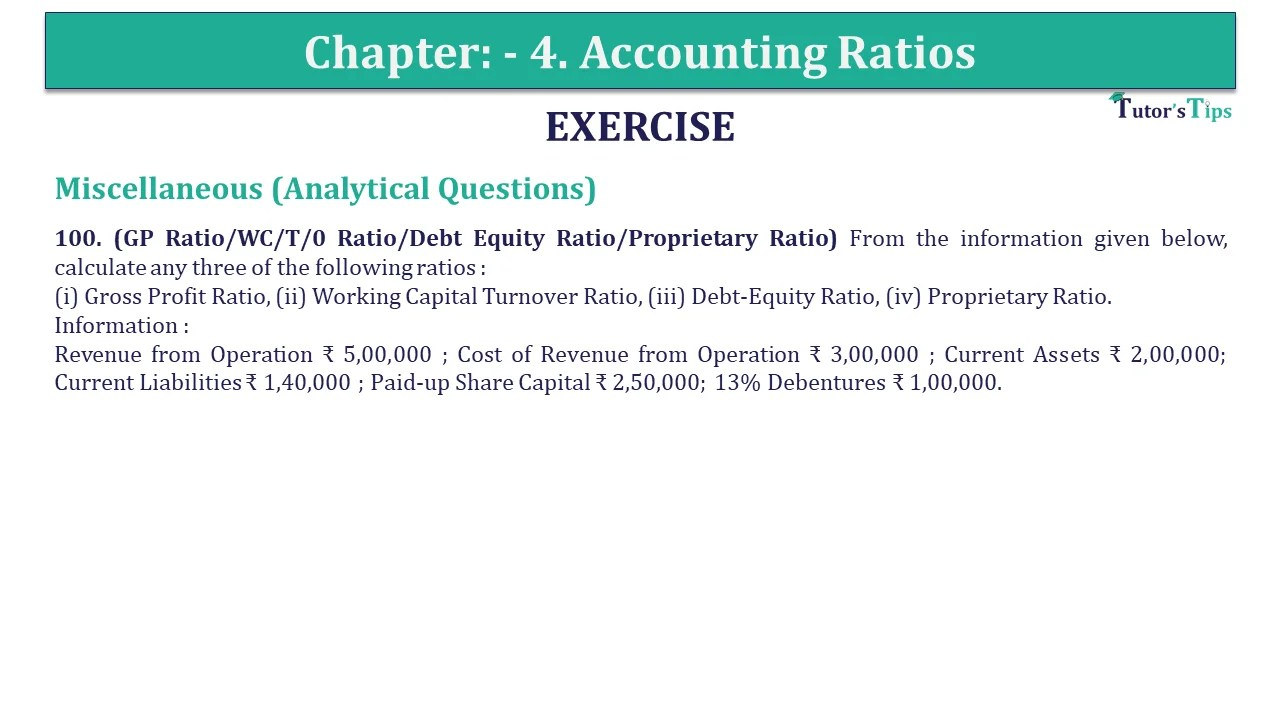# Question 100 Chapter 4 of +2-B – USHA Publication 12 ClassQ-100- CH-4 Book 2 - Usha Pub. +2 Book 2020 - Solution

Question 100 Chapter 4 of +2-B

Miscellaneous (Analytical Questions)

100. (GP Ratio/WC/T/0 Ratio/Debt Equity Ratio/Proprietary Ratio) From the information given below, calculate any three of the following ratios :
(i) Gross Profit Ratio, (ii) Working Capital Turnover Ratio, (iii) Debt-Equity Ratio, (iv) Proprietary Ratio.
Information :
Revenue from Operation ₹ 5,00,000 ; Cost of Revenue from Operation ₹ 3,00,000 ; Current Assets ₹ 2,00,000; Current Liabilities ₹ 1,40,000 ; Paid-up Share Capital ₹ 2,50,000; 13% Debentures ₹ 1,00,000.

## The solution of Question 100 Chapter 4 of +2-B: –

 (a) Gross Profit Ratio = Gross Profit X 100 Net Sales = = ₹ 2,00,000 X 100 ₹ 5,00,000 = 40%

 (ii) Working Capital Turnover Ratio = Net Sales Working Capital = = ₹ 5,00,000 ₹ 60,000 = 8.33 times
 (iii) Debt Equity Ratio = Long Term Debts Shareholders Funds = = ₹ 1,00,000 ₹ 2,50,000 = 0.4 : 1

 (iv) Proprietary Ratio = Proprietor’s Funds Total Assets = = ₹ 2,50,000 ₹ 4,90,000 = 51%
 Gross Profit = Net Sales – Cost of goods sold = ₹ 5,00,000 – ₹ 3,00,000 = ₹ 2,00,000 Working Capital = Current Assets – Current Liabilities = ₹ 2,00,000 – ₹ 1,40,000 = ₹ 60,000 Total Assets = Share capital + Current Liabilities + 13% Debentures = ₹ 2,50,000 + ₹ 1,40,000 + ₹ 1,00,000 = ₹ 4,90,000

Also, Check out the solved question of previous Chapters: –